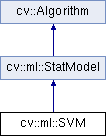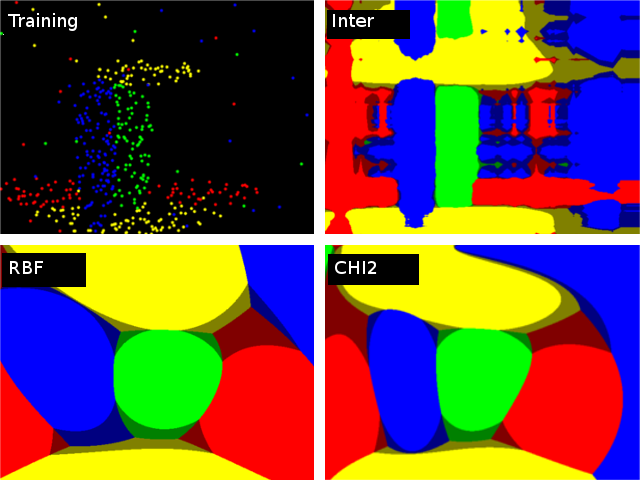OpenCV  3.0.0 Open Source Computer Vision
cv::ml::SVM Class Referenceabstract

Support Vector Machines. More...

#include "ml.hpp"

Inheritance diagram for cv::ml::SVM:class  Kernel

## Public Types

enum  KernelTypes {
CUSTOM =-1,
LINEAR =0,
POLY =1,
RBF =2,
SIGMOID =3,
CHI2 =4,
INTER =5
}
SVM kernel type More...

enum  ParamTypes {
C =0,
GAMMA =1,
P =2,
NU =3,
COEF =4,
DEGREE =5
}
SVM params type More...

enum  Types {
C_SVC =100,
NU_SVC =101,
ONE_CLASS =102,
EPS_SVR =103,
NU_SVR =104
}
SVM type More...Public Types inherited from cv::ml::StatModel
enum  Flags {
UPDATE_MODEL = 1,
RAW_OUTPUT =1,
COMPRESSED_INPUT =2,
PREPROCESSED_INPUT =4
}

## Public Member Functions

virtual double getC () const =0

virtual cv::Mat getClassWeights () const =0

virtual double getCoef0 () const =0

virtual double getDecisionFunction (int i, OutputArray alpha, OutputArray svidx) const =0
Retrieves the decision function. More...

virtual double getDegree () const =0

virtual double getGamma () const =0

virtual int getKernelType () const =0

virtual double getNu () const =0

virtual double getP () const =0

virtual Mat getSupportVectors () const =0
Retrieves all the support vectors. More...

virtual cv::TermCriteria getTermCriteria () const =0

virtual int getType () const =0

virtual void setC (double val)=0

virtual void setClassWeights (const cv::Mat &val)=0

virtual void setCoef0 (double val)=0

virtual void setCustomKernel (const Ptr< Kernel > &_kernel)=0

virtual void setDegree (double val)=0

virtual void setGamma (double val)=0

virtual void setKernel (int kernelType)=0

virtual void setNu (double val)=0

virtual void setP (double val)=0

virtual void setTermCriteria (const cv::TermCriteria &val)=0

virtual void setType (int val)=0

virtual bool trainAuto (const Ptr< TrainData > &data, int kFold=10, ParamGrid Cgrid=SVM::getDefaultGrid(SVM::C), ParamGrid gammaGrid=SVM::getDefaultGrid(SVM::GAMMA), ParamGrid pGrid=SVM::getDefaultGrid(SVM::P), ParamGrid nuGrid=SVM::getDefaultGrid(SVM::NU), ParamGrid coeffGrid=SVM::getDefaultGrid(SVM::COEF), ParamGrid degreeGrid=SVM::getDefaultGrid(SVM::DEGREE), bool balanced=false)=0
Trains an SVM with optimal parameters. More...Public Member Functions inherited from cv::ml::StatModel
virtual float calcError (const Ptr< TrainData > &data, bool test, OutputArray resp) const
Computes error on the training or test dataset. More...

virtual bool empty () const
Returns true if the Algorithm is empty (e.g. in the very beginning or after unsuccessful read. More...

virtual int getVarCount () const =0
Returns the number of variables in training samples. More...

virtual bool isClassifier () const =0
Returns true if the model is classifier. More...

virtual bool isTrained () const =0
Returns true if the model is trained. More...

virtual float predict (InputArray samples, OutputArray results=noArray(), int flags=0) const =0
Predicts response(s) for the provided sample(s) More...

virtual bool train (const Ptr< TrainData > &trainData, int flags=0)
Trains the statistical model. More...

virtual bool train (InputArray samples, int layout, InputArray responses)
Trains the statistical model. More...Public Member Functions inherited from cv::Algorithm
Algorithm ()

virtual ~Algorithm ()

virtual void clear ()
Clears the algorithm state. More...

virtual String getDefaultName () const

virtual void read (const FileNode &fn)
Reads algorithm parameters from a file storage. More...

virtual void save (const String &filename) const

virtual void write (FileStorage &fs) const
Stores algorithm parameters in a file storage. More...

## Static Public Member Functions

static Ptr< SVMcreate ()

static ParamGrid getDefaultGrid (int param_id)
Generates a grid for SVM parameters. More...Static Public Member Functions inherited from cv::ml::StatModel
template<typename _Tp >
static Ptr< _Tp > train (const Ptr< TrainData > &data, int flags=0)
Create and train model with default parameters. More...Static Public Member Functions inherited from cv::Algorithm
template<typename _Tp >
static Ptr< _Tp > load (const String &filename, const String &objname=String())
Loads algorithm from the file. More...

template<typename _Tp >
static Ptr< _Tp > loadFromString (const String &strModel, const String &objname=String())
Loads algorithm from a String. More...

template<typename _Tp >
static Ptr< _Tp > read (const FileNode &fn)
Reads algorithm from the file node. More...

## Detailed Description

Support Vector Machines.

Support Vector Machines

## Member Enumeration Documentation

SVM kernel type

A comparison of different kernels on the following 2D test case with four classes. Four SVM::C_SVC SVMs have been trained (one against rest) with auto_train. Evaluation on three different kernels (SVM::CHI2, SVM::INTER, SVM::RBF). The color depicts the class with max score. Bright means max-score > 0, dark means max-score < 0.image
Enumerator
CUSTOM

Returned by SVM::getKernelType in case when custom kernel has been set

LINEAR

Linear kernel. No mapping is done, linear discrimination (or regression) is done in the original feature space. It is the fastest option. $$K(x_i, x_j) = x_i^T x_j$$.

POLY

Polynomial kernel: $$K(x_i, x_j) = (\gamma x_i^T x_j + coef0)^{degree}, \gamma > 0$$.

RBF

Radial basis function (RBF), a good choice in most cases. $$K(x_i, x_j) = e^{-\gamma ||x_i - x_j||^2}, \gamma > 0$$.

SIGMOID

Sigmoid kernel: $$K(x_i, x_j) = \tanh(\gamma x_i^T x_j + coef0)$$.

CHI2

Exponential Chi2 kernel, similar to the RBF kernel: $$K(x_i, x_j) = e^{-\gamma \chi^2(x_i,x_j)}, \chi^2(x_i,x_j) = (x_i-x_j)^2/(x_i+x_j), \gamma > 0$$.

INTER

Histogram intersection kernel. A fast kernel. $$K(x_i, x_j) = min(x_i,x_j)$$.

SVM params type

Enumerator
GAMMA
NU
COEF
DEGREE
 enum cv::ml::SVM::Types

SVM type

Enumerator
C_SVC

C-Support Vector Classification. n-class classification (n $$\geq$$ 2), allows imperfect separation of classes with penalty multiplier C for outliers.

NU_SVC

$$\nu$$-Support Vector Classification. n-class classification with possible imperfect separation. Parameter $$\nu$$ (in the range 0..1, the larger the value, the smoother the decision boundary) is used instead of C.

ONE_CLASS

Distribution Estimation (One-class SVM). All the training data are from the same class, SVM builds a boundary that separates the class from the rest of the feature space.

EPS_SVR

$$\epsilon$$-Support Vector Regression. The distance between feature vectors from the training set and the fitting hyper-plane must be less than p. For outliers the penalty multiplier C is used.

NU_SVR

$$\nu$$-Support Vector Regression. $$\nu$$ is used instead of p. See  for details.

## Member Function Documentation

 static Ptr cv::ml::SVM::create ( )
static

Creates empty model. Use StatModel::train to train the model. Since SVM has several parameters, you may want to find the best parameters for your problem, it can be done with SVM::trainAuto.

 virtual double cv::ml::SVM::getC ( ) const
pure virtual

Parameter C of a SVM optimization problem. For SVM::C_SVC, SVM::EPS_SVR or SVM::NU_SVR. Default value is 0.

setC
 virtual cv::Mat cv::ml::SVM::getClassWeights ( ) const
pure virtual

Optional weights in the SVM::C_SVC problem, assigned to particular classes. They are multiplied by C so the parameter C of class i becomes classWeights(i) * C. Thus these weights affect the misclassification penalty for different classes. The larger weight, the larger penalty on misclassification of data from the corresponding class. Default value is empty Mat.

setClassWeights
 virtual double cv::ml::SVM::getCoef0 ( ) const
pure virtual

Parameter coef0 of a kernel function. For SVM::POLY or SVM::SIGMOID. Default value is 0.

setCoef0
 virtual double cv::ml::SVM::getDecisionFunction ( int i, OutputArray alpha, OutputArray svidx ) const
pure virtual

Retrieves the decision function.

Parameters
 i the index of the decision function. If the problem solved is regression, 1-class or 2-class classification, then there will be just one decision function and the index should always be 0. Otherwise, in the case of N-class classification, there will be $$N(N-1)/2$$ decision functions. alpha the optional output vector for weights, corresponding to different support vectors. In the case of linear SVM all the alpha's will be 1's. svidx the optional output vector of indices of support vectors within the matrix of support vectors (which can be retrieved by SVM::getSupportVectors). In the case of linear SVM each decision function consists of a single "compressed" support vector.

The method returns rho parameter of the decision function, a scalar subtracted from the weighted sum of kernel responses.

 static ParamGrid cv::ml::SVM::getDefaultGrid ( int param_id )
static

Generates a grid for SVM parameters.

Parameters
 param_id SVM parameters IDs that must be one of the SVM::ParamTypes. The grid is generated for the parameter with this ID.

The function generates a grid for the specified parameter of the SVM algorithm. The grid may be passed to the function SVM::trainAuto.

 virtual double cv::ml::SVM::getDegree ( ) const
pure virtual

Parameter degree of a kernel function. For SVM::POLY. Default value is 0.

setDegree
 virtual double cv::ml::SVM::getGamma ( ) const
pure virtual

Parameter $$\gamma$$ of a kernel function. For SVM::POLY, SVM::RBF, SVM::SIGMOID or SVM::CHI2. Default value is 1.

setGamma
 virtual int cv::ml::SVM::getKernelType ( ) const
pure virtual

Type of a SVM kernel. See SVM::KernelTypes. Default value is SVM::RBF.

 virtual double cv::ml::SVM::getNu ( ) const
pure virtual

Parameter $$\nu$$ of a SVM optimization problem. For SVM::NU_SVC, SVM::ONE_CLASS or SVM::NU_SVR. Default value is 0.

setNu
 virtual double cv::ml::SVM::getP ( ) const
pure virtual

Parameter $$\epsilon$$ of a SVM optimization problem. For SVM::EPS_SVR. Default value is 0.

setP
 virtual Mat cv::ml::SVM::getSupportVectors ( ) const
pure virtual

Retrieves all the support vectors.

The method returns all the support vector as floating-point matrix, where support vectors are stored as matrix rows.

 virtual cv::TermCriteria cv::ml::SVM::getTermCriteria ( ) const
pure virtual

Termination criteria of the iterative SVM training procedure which solves a partial case of constrained quadratic optimization problem. You can specify tolerance and/or the maximum number of iterations. Default value is TermCriteria( TermCriteria::MAX_ITER + TermCriteria::EPS, 1000, FLT_EPSILON );

setTermCriteria
 virtual int cv::ml::SVM::getType ( ) const
pure virtual

Type of a SVM formulation. See SVM::Types. Default value is SVM::C_SVC.

setType
 virtual void cv::ml::SVM::setC ( double val )
pure virtual

getC
 virtual void cv::ml::SVM::setClassWeights ( const cv::Mat & val )
pure virtual

getClassWeights
 virtual void cv::ml::SVM::setCoef0 ( double val )
pure virtual

getCoef0
 virtual void cv::ml::SVM::setCustomKernel ( const Ptr< Kernel > & _kernel )
pure virtual

Initialize with custom kernel. See SVM::Kernel class for implementation details

 virtual void cv::ml::SVM::setDegree ( double val )
pure virtual

getDegree
 virtual void cv::ml::SVM::setGamma ( double val )
pure virtual

getGamma
 virtual void cv::ml::SVM::setKernel ( int kernelType )
pure virtual

Initialize with one of predefined kernels. See SVM::KernelTypes.

 virtual void cv::ml::SVM::setNu ( double val )
pure virtual

getNu
 virtual void cv::ml::SVM::setP ( double val )
pure virtual

getP
 virtual void cv::ml::SVM::setTermCriteria ( const cv::TermCriteria & val )
pure virtual

getTermCriteria
 virtual void cv::ml::SVM::setType ( int val )
pure virtual

getType
 virtual bool cv::ml::SVM::trainAuto ( const Ptr< TrainData > & data, int kFold = 10, ParamGrid Cgrid = SVM::getDefaultGrid(SVM::C), ParamGrid gammaGrid = SVM::getDefaultGrid(SVM::GAMMA), ParamGrid pGrid = SVM::getDefaultGrid(SVM::P), ParamGrid nuGrid = SVM::getDefaultGrid(SVM::NU), ParamGrid coeffGrid = SVM::getDefaultGrid(SVM::COEF), ParamGrid degreeGrid = SVM::getDefaultGrid(SVM::DEGREE), bool balanced = false )
pure virtual

Trains an SVM with optimal parameters.

Parameters
 data the training data that can be constructed using TrainData::create or TrainData::loadFromCSV. kFold Cross-validation parameter. The training set is divided into kFold subsets. One subset is used to test the model, the others form the train set. So, the SVM algorithm is executed kFold times. Cgrid grid for C gammaGrid grid for gamma pGrid grid for p nuGrid grid for nu coeffGrid grid for coeff degreeGrid grid for degree balanced If true and the problem is 2-class classification then the method creates more balanced cross-validation subsets that is proportions between classes in subsets are close to such proportion in the whole train dataset.

The method trains the SVM model automatically by choosing the optimal parameters C, gamma, p, nu, coef0, degree. Parameters are considered optimal when the cross-validation estimate of the test set error is minimal.

If there is no need to optimize a parameter, the corresponding grid step should be set to any value less than or equal to 1. For example, to avoid optimization in gamma, set gammaGrid.step = 0, gammaGrid.minVal, gamma_grid.maxVal as arbitrary numbers. In this case, the value Gamma is taken for gamma.

And, finally, if the optimization in a parameter is required but the corresponding grid is unknown, you may call the function SVM::getDefaultGrid. To generate a grid, for example, for gamma, call SVM::getDefaultGrid(SVM::GAMMA).

This function works for the classification (SVM::C_SVC or SVM::NU_SVC) as well as for the regression (SVM::EPS_SVR or SVM::NU_SVR). If it is SVM::ONE_CLASS, no optimization is made and the usual SVM with parameters specified in params is executed.

The documentation for this class was generated from the following file: